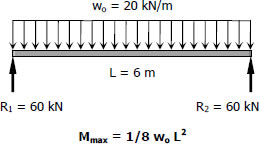# For Beams (B - 3) | Solution to Problem 542

## For Beams (B - 3)$M_{max} = \frac{1}{8}(20)(62)$
$M_{max} = 90 \, \text{kN}\cdot\text{m}$

$S_{required} = \dfrac{M_{max}}{f_b} = \dfrac{90(1000^2)}{120}$
$S_{required} = 750 \times 10^3 \, \text{mm}^3$

From Appendix B, Table B-2 Properties of Wide Flange Sections (W Shapes): SI Units, of text book:

 Designation Section Modulus W200 × 86 853 × 103 mm3 W250 × 67 806 × 103 mm3 W310 × 60 849 × 103 mm3 W360 × 51 796 × 103 mm3 W410 × 46 773 × 103 mm3

Consider W410 × 46 with S = 773 × 103 mm3

From the Checking of B - 2
$S_{own-weight} = 7521 \, \text{mm}^3$
$S_{required} + S_{own-weight} = (750 \times 10^3) + 7521$
$S_{required} + S_{own-weight} = 757\,521 \, \text{mm}^3$

$(S_{supplied} = 773 \times 10^3 \, \text{mm}^3) \gt 757\,521 \, \text{mm}^3 \,\,$ (ok!)

Use W410 × 46 for B-3, this section is the same to B - 2. answer

Solution for Beams (B - 1)
Solution for Girder (G - 1)
Solution for Beams (B - 2)
Solution for Beams (B - 3)
Solution for Girders (G - 2)